### Polynomial Interpolation: Lagrange Interpolating Polynomials

### Lagrange Interpolating Polynomials

Another equivalent method to find the interpolating polynomials is using the Lagrange Polynomials. Given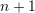data points: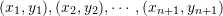, then the Lagrange polynomial of degreethat fits through thedata points has the form: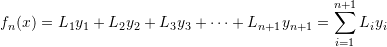where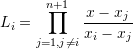In the following Mathematica code a Lagrange polynomial procedure is created to output the Lagrange polynomials.

View Mathematica Code
Data = {{1, 3}, {4, 5}, {5, 6}, {6, 8}, {7, 8}};
Lagrange[anydata_] := Sum[anydata[[i, 2]]*LP[anydata, i], {i, 1, Length[anydata]}]
LP[anydata_, i_] := (newdata = Drop[anydata, {i}]; Product[(x - newdata[[j, 1]])/(anydata[[i, 1]] - newdata[[j, 1]]), {j, 1, Length[newdata]}])
(*Using the Lagrange polynomial function*)
y = Expand[Lagrange[Data]]
(*Using the interpolating polynomial built-in function*)
y2 = Expand[InterpolatingPolynomial[Data, x]]

View Python Code
import numpy as np
import sympy as sp
sp.init_printing(use_latex=True)

x = sp.symbols('x')
Data = [(1, 3), (4, 5), (5, 6), (6, 8), (7, 8)]
def Lagrange(anydata):
return sum([anydata[i]*LP(anydata,i) for i in range(len(anydata))])
def LP(anydata, i):
newdata = anydata.copy()
newdata.pop(i)
return np.prod([(x - newdata[j])/(anydata[i] - newdata[j]) for j in range(len(newdata))])
# Using the Lagrange polynomial function
display("y:  ",sp.expand(Lagrange(Data)))
# Using the interpolating polynomial built-in function
display("y2: ",sp.interpolate(Data, x))


The Lagrange interpolating polynomials produce the same polynomial as the general method and the Newton’s interpolating polynomials. The examples used for the Newton’s interpolating polynomials will be repeated here.

#### Example 1

Using Lagrange interpolating polynomials, find the interpolating polynomial to the data: (1,1),(2,5).

##### Solution

We have two data points, so, we will create a polynomial of the first degree. Therefore, the interpolating polynomial has the form: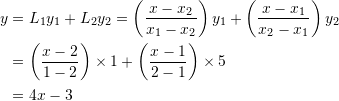#### Example 2

Using Lagrange interpolating polynomials, find the interpolating polynomial to the data: (1,1),(2,5),(3,2).

##### Solution

We have three data points, so, we will create a polynomial of the second degree. Using Lagrange polynomials: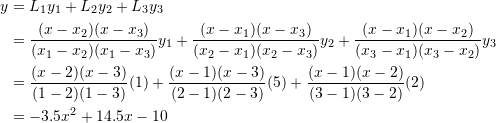#### Example 3

Using Lagrange polynomials, find the interpolating polynomial to the data: (1,1),(2,5),(3,2),(3.2,7),(3.9,4).

##### Solution

A fourth order polynomial would be needed to pass through five data points. Using Lagrange polynomials, the required function has the form: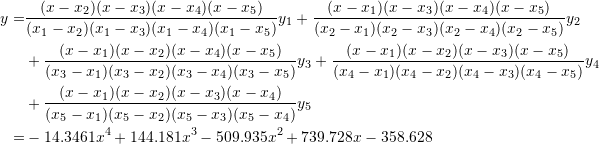MATLAB file for the above examples: